Courses

# NCERT Solutions - Work and Energy Class 9 Notes | EduRev

## UPSC : NCERT Solutions - Work and Energy Class 9 Notes | EduRev

The document NCERT Solutions - Work and Energy Class 9 Notes | EduRev is a part of the UPSC Course NCERT Textbooks (Class 6 to Class 12).
All you need of UPSC at this link: UPSC

Page No. 148

Q.1. A force of 7 N acts on an object. The displacement is, say 8 m, in the direction of the force (fig given). Let us take it that the force acts on the object through the displacement. What is the work done in this case?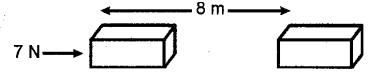Ans. When a force F acts on an object to displace it through a distance S in its direction, then the work done W on the body by the force is given by:
Work was done = Force × Displacement
W = F × S
Where:
F = 7 N
S = 8 m
Therefore, work done, W = 7 × 8
= 56 Nm
= 56 J

Page No. 149

Q.1. When do we say that work is done?

Ans. Work is done whenever the given conditions are satisfied:

• A force acts on the body.
• There is a displacement of the body caused by the applied force along the direction of the applied force.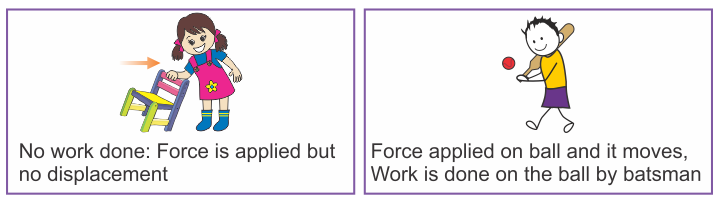Q.2. Write an expression for the work done when a force is acting on an object in the direction of its displacement.
Ans. When a force F displaces a body through a distance S in the direction of the applied force, then the work done W on the body is given by the expression:
Work was done = Force × Displacement
W = F × s

Q.3. Define 1 J of work.
Ans. 1 J is the amount of work done by a force of 1 N on an object that displaces it through a distance of 1 m in the direction of the applied force.

Q.4. A pair of bullocks exerts a force of 140 N on a plough. The field being ploughed is 15 m long. How much work is done in ploughing the length of the field?
Ans. Work done by the bullocks is given by the expression:
Work was done = Force × Displacement
W = F × d
Where:
Applied force, F = 140 N
Displacement, d = 15 m
W = 140 × 15 = 2100 J
Hence, 2100 J of work is done in ploughing the length of the field.

Page No. 152

Q.1. What is the kinetic energy of an object?
Ans.

• Kinetic energy is the energy possessed by a body by virtue of its motion. Every moving object possesses kinetic energy.
• A body uses kinetic energy to do work. The kinetic energy of hammer is used in driving a nail into a log of wood, kinetic energy of air is used to run windmills, etc.

Q.2. Write an expression for the kinetic energy of an object.
Ans. If a body of mass m is moving with a velocity v, then its kinetic energy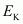is given by the expression: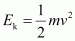Its SI unit is Joule (J).

Q.3. The kinetic energy of an object of mass, m moving with a velocity of 5 ms-1 is 25 J. What will be its kinetic energy when its velocity is doubled? What will be its kinetic energy when its velocity is increased three times?
Ans. Expression for kinetic energy ism = Mass of the object
v = Velocity of the object = 5 ms−1
Given that kinetic energy,= 25 J

• If the velocity of an object is doubled, then v = 5 × 2 = 10 m s−1.
Therefore, its kinetic energy becomes 4 times its original value, because it is proportional to the square of the velocity. Hence, kinetic energy = 25 × 4 = 100 J.
• If velocity is increased three times, then its kinetic energy becomes 9 times its original value, because it is proportional to the square of the velocity. Hence, kinetic energy = 25 × 9 = 225 J.
Page No. 156

Q.1. What is power?
Ans. Power is the rate of doing work or the rate of transfer of energy. If 'W' is the amount of work done in time 't', then power is given by the expression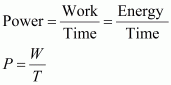It is expressed in watt (W).

Q.2. Define 1 watt of power.
Ans. A body is said to have power of 1 watt if it does work at the rate of 1 joule in 1 s, i.e.,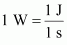Q.3. A lamp consumes 1000J of electrical energy in 10s. What is its power?
Ans. Power is given by the expression: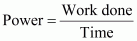Work done = Energy consumed by the lamp = 1000 J
Time = 10 s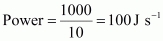= 100 W

Q.4. Define average power.
Ans. A body can do different amount of work at different time intervals. Hence, it is better to define average power. Average power is obtained by dividing the total amount of work done in the total time taken to do this work.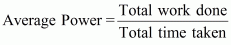Page No. 158

Q.1. Look at the activities listed below. Reason out whether or not work is done in the light of your understanding of the term ‘work’.

• Suma is swimming in a pond.
• A donkey is carrying a load on its back.
• A windmill is lifting water from a well.
• A green plant is carrying out photosynthesis.
• An engine is pulling a train.
• Food grains are getting dried in the sun.
• A sailboat is moving due to wind energy.

Ans. (i) Suma is swimming in a pond: In this case, Suma pushes the water in the backward direction. It is known as action. However, due to reaction, the water pushes the person in the forward direction. In this situation, the force and displacement are in the same direction. The work is done by Suma and the work done by the force is positive.
(ii) A donkey is carrying a load on its back: If the displacement of an object is perpendicular to the force acting on it, the work done by the force on the object is zero.
In the given situation, the force of gravity on the load is in the downward direction, whereas the displacement is in the horizontal direction, i.e., the force and the displacement are perpendicular to each other. There is no displacement in the direction of the force of gravity, and therefore, work done is zero.
(iii) A windmill is lifting water from a well: In this situation, the object (bucket of water from a well) moves upwards. The force exerted by windmill is in the direction of displacement. Therefore, work is done. This work done is positive as the force and the displacement are in same direction.
(iv) A green plant is carrying out photosynthesis: No work is done in this case.
(v) An engine is pulling a train: In this situation, an engine is pulling a train parallel to the ground. The force exerted by the engine is in the direction of displacement of the train. Thus, the force and the displacement are in the same direction. Therefore, work is done. This work done is positive.
(vi) Foodgrains are getting dried in the sun: No work is done in this case.
(vii) A sailboat moving due to wind energy: The force exerted by wind on the sail move the boat in the directions of force, hence, positive work is done by wind energy.

Q.2. An object thrown at a certain angle to the ground moves in a curved path and falls back to the ground. The initial and the final points of the path of the object lie on the same horizontal line. What is the work done by the force of gravity on the object?
Ans.
Work done by the force of gravity on an object depends only on vertical displacement. Vertical displacement is given by the difference in the initial and final positions/heights of the object, which is zero.
Work done by gravity is given by the expression:
W = mgh
Where:
h = Vertical displacement = 0
W = mg × 0 = 0 J
Therefore, the work done by gravity on the given object is zero joule.

Q.3. A battery lights a bulb. Describe the energy changes involved in the process.
Ans.

• When a bulb is connected to a battery, then the chemical energy of the battery is transferred into electrical energy.
• When the bulb receives this electrical energy, then it converts it into light and heat energy.
• Hence, the transformation of energy in the given situation can be shown as: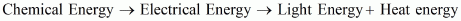Q.4. Certain force acting on a 20kg mass changes its velocity from 5ms−1 to 2m s−1. Calculate the work done by the force.
Ans. Kinetic energy is given by the expression: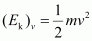Where: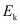= Kinetic energy of the object moving with a velocity, v
m = Mass of the object
(i) Kinetic energy when the object was moving with a velocity 5ms−1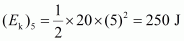Kinetic energy when the object was moving with a velocity 2ms−1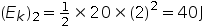Work done by force is equal to the change in kinetic energy.
Therefore, work done by force =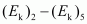= 40 − 250 = −210 J

The negative sign indicates that the force is acting in the direction opposite to the motion of the object.

Q.5. A mass of 10 kg is at a point A on a table. It is moved to a point B. If the line joining A and B is horizontal, what is the work done on the object by the gravitational force? Explain your answer.
Ans. Work done by gravity depends only on the vertical displacement of the body. It does not depend upon the path of the body.
Therefore, work done by gravity is given by the expression:
W = mgh
Where:
Vertical displacement, h = 0
W = mg × 0 = 0
Hence, the work done by gravity on the body is zero.

Q.6. The potential energy of a freely falling object decreases progressively. Does this violate the law of conservation of energy? Why?
Ans.

• No. The process does not violate the law of conservation of energy. This is because when the body falls from a height, then its potential energy changes into kinetic energy progressively.
• A decrease in the potential energy is equal to an increase in the kinetic energy of the body.
• During the process, total mechanical energy of the body remains conserved. Therefore, the law of conservation of energy is not violated.

Q.7. What are the various energy transformations that occur when you are riding a bicycle?
Ans.

• While riding a bicycle, the muscular energy of the rider gets transferred into heat energy and kinetic energy of the bicycle.
• Heat energy heats the rider’s body. Kinetic energy provides a velocity to the bicycle.
The transformation can be shown as: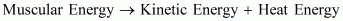During the transformation, the total energy remains conserved.

Q.8. Does the transfer of energy take place when you push a huge rock with all your might and fail to move it? Where is the energy you spend going?
Ans. When we push a huge rock, there is no transfer of muscular energy to the stationary rock. Also, there is no loss of energy because muscular energy is transferred into heat energy, which causes our body to become hot.

Q.9. A certain household has consumed 250 units of energy during a month. How much energy is this in joules?
Ans. 1 unit of energy is equal to 1 kilowatt-hour (kWh).
1 unit = 1 kWh
1 kWh = 3.6 × 106 J
Therefore, 250 units of energy = 250 × 3.6 × 106 = 9 × 108 J

Q.10. An object of mass 40 kg is raised to a height of 5 m above the ground. What is its potential energy? If the object is allowed to fall, find its kinetic energy when it is half-way down.
Ans. Gravitational potential energy is given by the expression:
W = mgh
Where:
h = Vertical displacement = 5 m
m = Mass of the object = 40 kg
g = Acceleration due to gravity = 9.8 m s−2
∴ W = 40 × 5 × 9.8 = 1960 J.
At half-way down, the potential energy of the object will be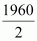= 980 J.
At this point, the object has an equal amount of potential and kinetic energy. This is due to the law of conservation of energy. Hence, half-way down, the kinetic energy of the object will be 980 J.

Q.11.  What is the work done by the force of gravity on a satellite moving round the earth? Justify your answer.
Ans. Work is done whenever the given two conditions are satisfied:
(i) A force acts on the body.
(ii) There is a displacement of the body by the application of force in or opposite to the direction of force.

• If the direction of force is perpendicular to displacement, then the work done is zero.
When a satellite moves around the Earth, then the direction of force of gravity on the satellite is perpendicular to its displacement. Hence, the work done on the satellite by the Earth is zero.

Q.12. Can there be displacement of an object in the absence of any force acting on it? Think. Discuss this question with your friends and teacher.
Ans. Yes. For a uniformly moving object.

• Suppose an object is moving with constant velocity. The net force acting on it is zero. But, there is a displacement along the motion of the object. Hence, there can be a displacement without a force.

Q.13. A person holds a bundle of hay over his head for 30 minutes and gets tired. Has he done some work or not? Justify your answer.
Ans. Work is done whenever the given two conditions are satisfied:
(i) A force acts on the body.
(ii) There is a displacement of the body by the application of force in or opposite to the direction of force.

• When a person holds a bundle of hay over his head, then there is no displacement in the bundle of hay. Although the force of gravity is acting on the bundle, the person is not applying any force on it. Hence, in the absence of force, work done by the person on the bundle is zero.

Q.14. An electric heater is rated 1500 W. How much energy does it use in 10 hours?
Ans. Energy consumed by an electric heater can be obtained with the help of the expression: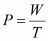Where:
Power rating of the heater, P = 1500 W = 1.5 kW
Time for which the heater has operated, T = 10 h
Work done = Energy consumed by the heater
Therefore, energy consumed = Power × Time
= 1.5 × 10 = 15 kWh
Hence, the energy consumed by the heater in 10h is 15 kWh.

Q.15. Illustrate the law of conservation of energy by discussing the energy changes which occur when we draw a pendulum bob to one side and allow it to oscillate. Why does the bob eventually come to rest? What happens to its energy eventually? Is it a violation of the law of conservation of energy?
Ans. The law of conservation of energy states that energy can neither be created nor destroyed. It can only be converted from one form to another.
Consider the case of an oscillating pendulum: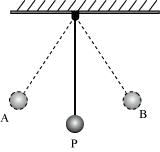• When a pendulum moves from its mean position P to either of its extreme positions A or B, it rises through a height h above the mean level P. At this point, the kinetic energy of the bob changes completely into potential energy. The kinetic energy becomes zero, and the bob possesses only potential energy.
• As it moves towards point P, its potential energy decreases progressively. Accordingly, the kinetic energy increases.
• As the bob reaches point P, its potential energy becomes zero and the bob possesses only kinetic energy. This process is repeated as long as the pendulum oscillates.
• The bob does not oscillate forever. It comes to rest because air resistance resists its motion.
• The pendulum loses its kinetic energy to overcome this friction and stops after some time.
• The law of conservation of energy is not violated because the energy lost by the pendulum to overcome friction is gained by its surroundings. Hence, the total energy of the pendulum and the surrounding system remain conserved.

Q.16. An object of mass, m is moving with a constant velocity, v. How much work should be done on the object in order to bring the object to rest?
Ans. Kinetic energy of an object of mass, m moving with a velocity, v is given by the expression:To bring the object to rest,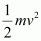amount of work is required to be done on the object.

Q.17. Calculate the work required to be done to stop a car of 1500 kg moving at a velocity of 60 km/h?
Ans. Kinetic energy,Where:
Mass of car, m = 1500 kg
Velocity of car, v = 60 km/h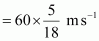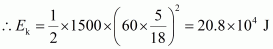Hence, 20.8 × 104 J of work is required to stop the car.

Q.18. In each of the following a force, 'F' is acting on an object of mass, 'm'. The direction of displacement is from west to east shown by the longer arrow. Observe the diagrams carefully and state whether the work done by the force is negative, positive or zero.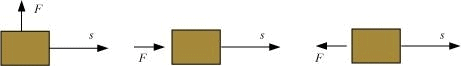Ans. Work is done whenever the given two conditions are satisfied:
(i) A force acts on the body.
(ii) There is a displacement of the body by the application of force in or opposite to the direction of force.
Case I: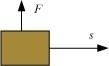In this case, the direction of force acting on the block is perpendicular to the displacement. Therefore, work done by force on the block will be zero.
Case II: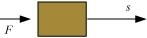In this case, the direction of force acting on the block is in the direction of displacement. Therefore, work done by force on the block will be positive.
Case III: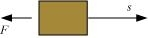In this case, the direction of force acting on the block is opposite to the direction of displacement. Therefore, work done by force on the block will be negative.

Q.19. Soni says that the acceleration in an object could be zero even when several forces are acting on it. Do you agree with her? Why?
Ans. Acceleration in an object could be zero even when several forces are acting on it. This happens when all the forces cancel out each other, i.e., the net force acting on the object is zero. For a uniformly moving object, the net force acting on the object is zero. Hence, the acceleration of the object is zero. Hence, Soni is right.

Q.20. Find the energy in kW h consumed in 10 hours by four devices of power 500 W each.
Ans. Energy consumed by an electric device can be obtained with the help of the expression for power:Where:
Power rating of the device, P = 500 W = 0.50 kW
Time for which the device runs, T = 10 h
Work done = Energy consumed by the device
Therefore, energy consumed = Power × Time
= 0.50 × 10 = 5 kWh
Hence, the energy consumed by four equal rating devices in 10 h will be 4 × 5 kWh = 20 kWh = 20 Units.

Q.21. A freely falling object eventually stops on reaching the ground. What happens to its kinetic energy?
Ans.

• When an object falls freely towards the ground, its potential energy decreases and kinetic energy increases.
• As the object touches the ground, all its potential energy gets converted into kinetic energy.
• As the object hits the hard ground, all its kinetic energy gets converted into heat energy and sound energy. It can also deform the ground depending upon the nature of the ground and the amount of kinetic energy possessed by the object.

Offer running on EduRev: Apply code STAYHOME200 to get INR 200 off on our premium plan EduRev Infinity!

## NCERT Textbooks (Class 6 to Class 12)

954 docs|585 tests

,

,

,

,

,

,

,

,

,

,

,

,

,

,

,

,

,

,

,

,

,

;